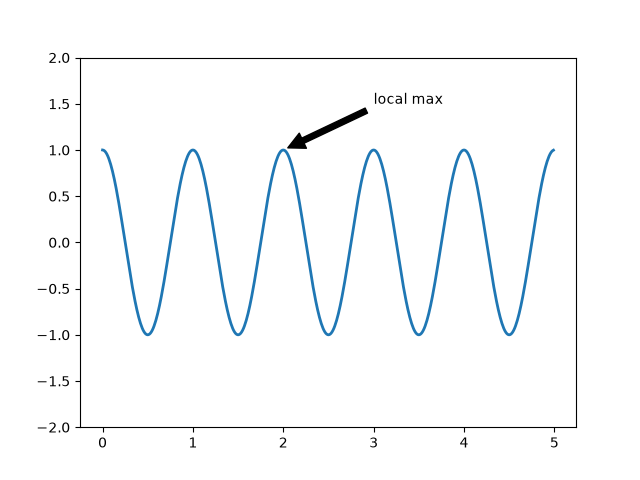# Annotating a plot#

This example shows how to annotate a plot with an arrow pointing to provided coordinates. We modify the defaults of the arrow, to "shrink" it.

For a complete overview of the annotation capabilities, also see the annotation tutorial.

import numpy as np
import matplotlib.pyplot as plt

fig, ax = plt.subplots()

t = np.arange(0.0, 5.0, 0.01)
s = np.cos(2*np.pi*t)
line, = ax.plot(t, s, lw=2)

ax.annotate('local max', xy=(2, 1), xytext=(3, 1.5),
arrowprops=dict(facecolor='black', shrink=0.05),
)
ax.set_ylim(-2, 2)
plt.show()References

The use of the following functions, methods, classes and modules is shown in this example:

Gallery generated by Sphinx-Gallery